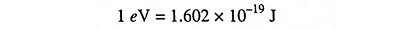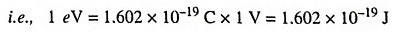## What is Electron Volt?

The joule (J) is the unit of energy in the SI system. In some engineering power problems this unit is very small, and a factor of 103 or 106 is introduced to convert from watts (1 W = 1 J/s) to kilowatts or megawatts, respectively. For a discussion of the energies involved in electronic devices, even the joule is too large a unit. This statement is not to be construed to mean that only minute amounts of energy can be obtained from electron devices. It is true that each electron possesses a tiny amount of energy, but an enormous number of electrons is involved even in a small current, so that considerable power may be represented. A unit of work or energy, called the electron volt (eV), is given asThe name electron volt arises from the fact that, if an electron falls through a potential of 1 V, its kinetic energy will increase by the decrease in potential energy, or by one electron volt.However, the eV unit may be used for any type of energy, and is not restricted to problems involving electrons.

The abbreviations MeV and BeV are used to designate 1 million and billion electron volts respectively.

Scroll to Top